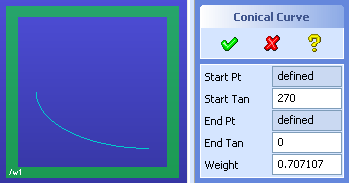> > Draw a conical curve

Draw a conical curve
Conical curves are based on the cross-section of a cone: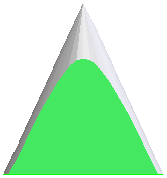Change the shape of a curve by adjusting its weight: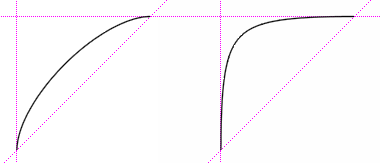To create a conical curve,
1. Click Modeling and then, in the Draw group, click the arrow next to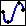Spline.
2. Click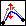Conical Curve in the Functional section. The Conical Curve dialog box opens.
3. Select a Start Point in the viewport or enter a coordinate in the User Input Line.
4. Select a Start Tangency in the viewport or enter an angle in the User Input Line.
5. Set the End Point and End Tangency like you set them for the start values.
6. Set the Weight in the viewport, enter a formula, or enter a coordinate.
7. Click OK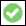to complete the operation.
Some interesting curves you can create
 Create a circular arc with a radius of 100:Start Pt: 0,100Start Tan: 270End Pt: 100,0End Tan: 0Weight: 1/sqrt(2)Use the Input Line to enter values for the start and end points. Enter the formula directly into the Weight field.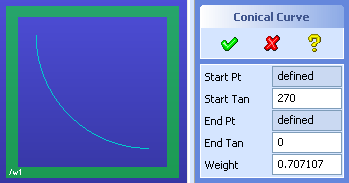Change the Weight to 1 and it becomes a parabolic curve: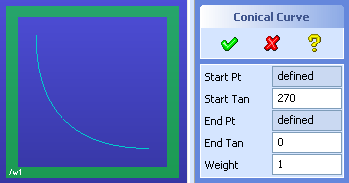Change the Start Pt to 0,50 and it becomes an elliptical arc with a major radius of 100 and a minor radius of 50: